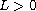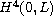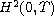Electron. J. Diff. Equ., Vol. 2015 (2015), No. 239, pp. 1-19.

### Boundary controllability for a nonlinear beam equation Xiao-Min Cao

Abstract:
This article concerns a nonlinear system modeling the bending vibrations of a nonlinear beam of length. First, we derive the existence of long time solutions near an equilibrium. Then we prove that the nonlinear beam is locally exact controllable around the equilibrium inand with control functions in. The approach we used are open mapping theorem, local controllability established by linearization, and the induction.

Submitted May 4, 2015. Published September 17, 2015.
Math Subject Classifications: 93B05, 35L75, 93C10, 93C20.
Key Words: Nonlinear beam equation; locally exact controllability; equilibrium; smooth control.

Show me the PDF file (277 KB), TEX file, and other files for this article.Xiao-Min Cao School of Mathematical Sciences Shanxi University Taiyuan 030006, China email: caoxm@sxu.edu.cn